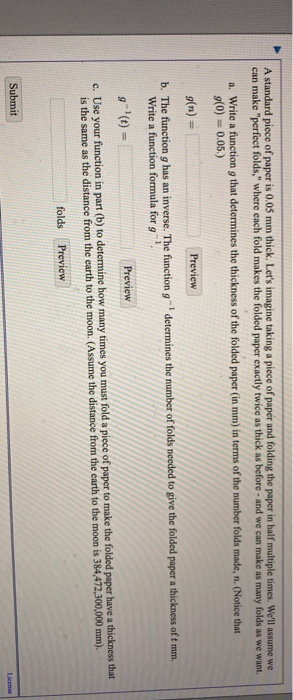# Question A standard piece of paper is 0.05 mm thick. Let's imagine taking a piece of paper and folding the paper in half multiple times. We'll assume we can make "perfect folds," where each fold makes the folded paper exactly twice as thick as before - and we can make as many folds as we want. a. Write a function g that determines the thickness of the folded paper (in mm) in terms of the number folds made, n. (Notice that 9(0) = 0.05.) g(n) Preview b. The function g has an inverse. The function - determines the number of folds needed to give the folded paper a thickness oft mm. Write a function formula for g 9-'(t) = Preview c. Use your function in part (b) to determine how many times you must fold a piece of paper to make the folded paper have a thickness that is the same as the distance from the earth to the moon. (Assume the distance from the earth to the moon is 384,472,300,000 mm). folds Preview Submit